## Divisor

A divisor of a numberis a number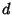which Divides, also called a Factor. The total number of divisors for a given numbercan be found as follows. Write a number in terms of its Prime Factorization(1)

For any divisorof,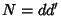where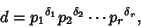(2)

so(3)

Now,, so there are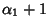possible values. Similarly, for, there arepossible values, so the total number of divisors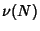ofis given by(4)

The functionis also sometimes denotedor. The product of divisors can be found by writing the numberin terms of all possible products(5)

so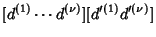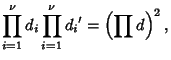(6)

and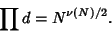(7)

The Geometric Mean of divisors is(8)

The sum of the divisors can be found as follows. Letwithand. For any divisorof,, whereis a divisor ofand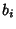is a divisor of. The divisors ofare 1,,, ..., and. The divisors ofare 1,,, ...,. The sums of the divisors are then(9)(10)

For a given,(11)

Summing over all,(12)

so. Splittingandinto prime factors,(13)

For a prime Power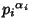, the divisors are 1,,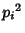, ...,, so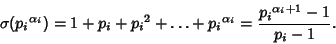(14)

For, therefore,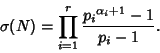(15)

For the special case ofa Prime, (15) simplifies to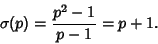(16)

Fora Power of two, (15) simplifies to(17)

The Arithmetic Mean is(18)

The Harmonic Mean is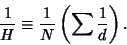(19)

But, so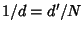and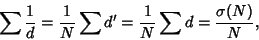(20)

and we have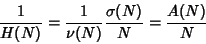(21)(22)

Given three Integers chosen at random, the probability that no common factor will divide them all is(23)

whereis Apéry's Constant.

Letbe the number of elements in the greatest subset of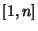such that none of its elements are divisible by two others. Forsufficiently large,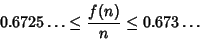(24)

(Le Lionnais 1983, Lebensold 1976/1977).

See also Aliquant Divisor, Aliquot Divisor, Aliquot Sequence, Dirichlet Divisor Problem, Divisor Function, e-Divisor, Exponential Divisor, Greatest Common Divisor, Infinary Divisor, k-ary Divisor, Perfect Number, Proper Divisor, Unitary Divisor

References

Guy, R. K. Solutions of.'' §B18 in Unsolved Problems in Number Theory, 2nd ed. New York: Springer-Verlag, pp. 73-75, 1994.

Le Lionnais, F. Les nombres remarquables. Paris: Hermann, p. 43, 1983.

Lebensold, K. A Divisibility Problem.'' Studies Appl. Math. 56, 291-294, 1976/1977.